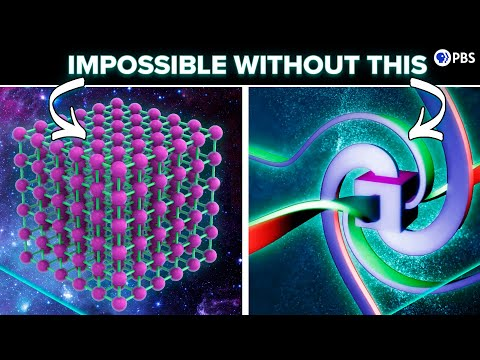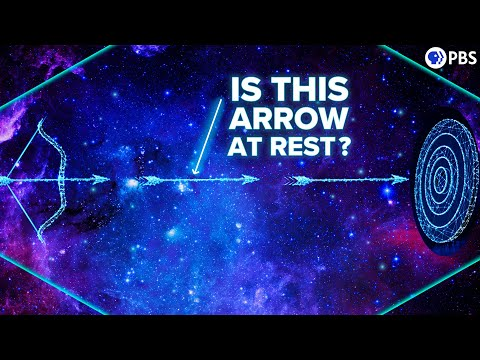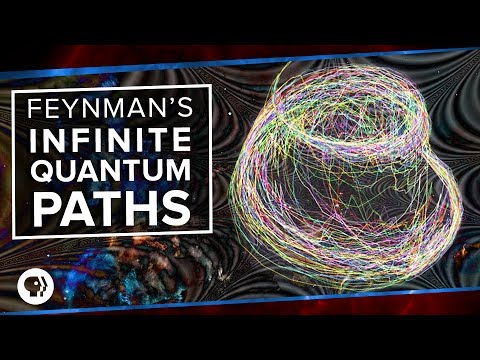# Search PBS Space Time

## Results### 2021-09-21: How Electron Spin Makes Matter Possible

• 08:00: ... location of the electron around the atom. Psi is really the so-called “probability amplitude”. ...### 2021-03-23: Zeno's Paradox & The Quantum Zeno Effect

• 02:58: ... to that observation, the arrow exists as just the distribution of probability amplitudes of its position; its wavefunction is spread between the start and end of ...### 2017-07-19: The Real Star Wars

• 17:07: In fact, the probability amplitude added at each vertex is proportional to the square root of the fine structure constant.
• 17:15: The probability itself is the square of the probability amplitude.### 2017-07-12: Solving the Impossible in Quantum Field Theory

• 06:50: It turns out that the probability amplitude of a particular interaction depends on the number of connections, or vertices, in the diagram.
• 14:10: [INAUDIBLE] points out that the final probability for a particle journey is the square of the length of the complex probability amplitude vector.
• 14:20: Probability is the square of the probability amplitude.
• 14:25: ... relay is that the total probability depends on the length of the summed probability amplitudes-- so the square of the real plus the square of the complex ...
• 14:37: ... also correctly points out that the individual paths don't have different probability amplitude lengths taken separately, but rather, they're pointing in the complex ...
• 15:06: ... applying the principle of least action in the determination of probability amplitudes, it turns out that the "crazy" paths, including the superluminal ones, ...### 2017-07-07: Feynman's Infinite Quantum Paths

• 05:50: Instead, each path contributes what we call a probability amplitude to the entire A-B journey.
• 05:58: Schrodinger's wave function and Feynman's path integral describe this probability amplitude thing.
• 06:10: However, probability amplitudes are what we call complex numbers.
• 06:15: We can think of each path's probability amplitude as a vector or an arrow of a certain length and direction in an imaginary 2D space.
• 06:32: But to get the total probability that a particle travels from A to B, you connect the probability amplitude arrows for all possible paths end to end.
• 06:52: But if two parts contribute individual probability amplitudes that point in exactly opposite directions, they cancel each other out.
• 07:13: Now, when Feynman used this action quantity to figure out the probability amplitudes of his infinite paths, something amazing happened.
• 10:04: The quantum action principle gives the probability amplitude of changes in the state of the field.
• 10:10: ... includes motion of the photon, but it also includes the probability amplitude of a photon's energy moving from the electromagnetic field into, say, ...
5 result(s) shown.